# Radial stress in plane strain 2D cylinder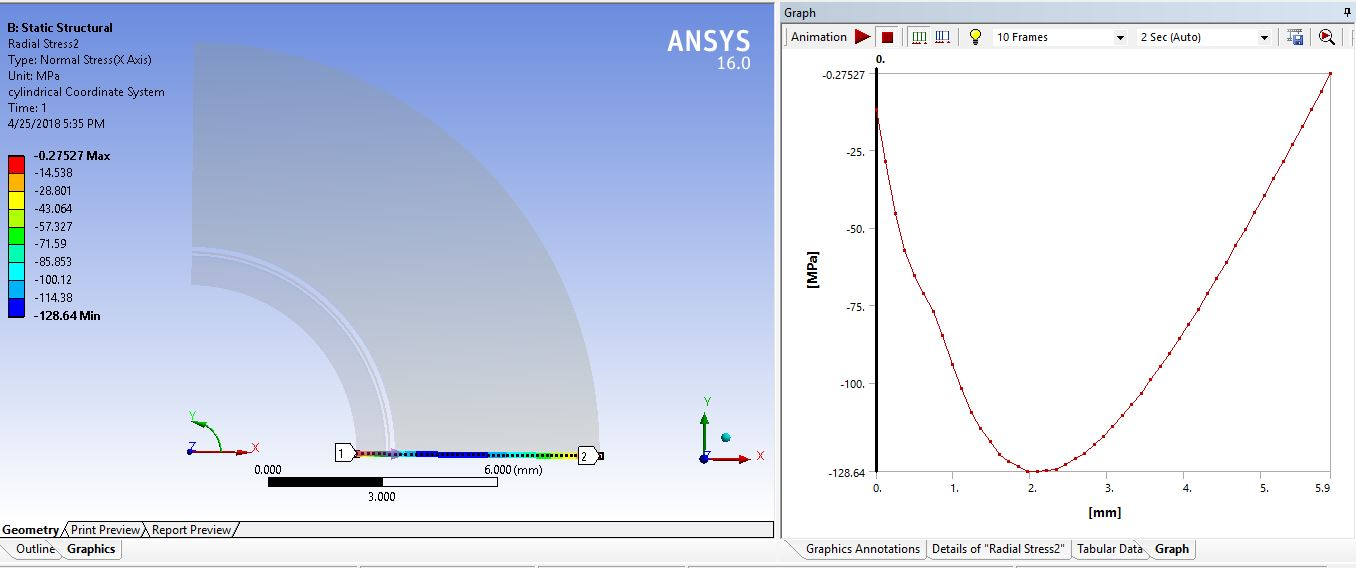How can i get Residual radial stress at room temperature, after the 1000 C temperature was applied and removed in a plane stress or plane strain 2D cylinder. I can get the radial stresses only but don't know how to get the residual radial stress from the post processing. I have attached the archive file for better understanding to help.

«1

• There are two ways to get residual stress in your problem.

One is where different materials are bonded at 1000 C without stress, then cooled to room temperature (22 C) and elastic residual stress is created due to the difference in CTE between the materials. If the material were reheated to 1000 C, the stress would again be zero. If this is your case, please confirm.

Another way is when the different materials were bonded at room temperature, so have no residual stress at 22 C, but at least one material passes its yield point as the temperature is raised to 1000 C and lowered again. This could result in permanent plastic deformation (or crushing if a porous material) and there would be a residual stress when the material returns to room temperature. If this is your case, your model has no material properties for plasticity or any nonlinear behavior.

A model could have both elastic and plastic causes of residual stress, so even if it was bonded at 1000 C, one material could pass its yield point as it cools to 22 C, which would be a different model and result from the first case.

Regards

• Sir peteroznewman,

First of all I am very happy to see your reply.I have been always looking for you when i am getting some mechanical workbench problem.I would like to learn the both ways to get the residual stresses sir.

If i provide the adhesive strength and,Hardness,Toughness and melting point then will it fulfill the demand for plastic properties?

Another thing i can give the numerical solution of the graph (deformation vs distance) of this model.If it helps you to consider the conditions and others on.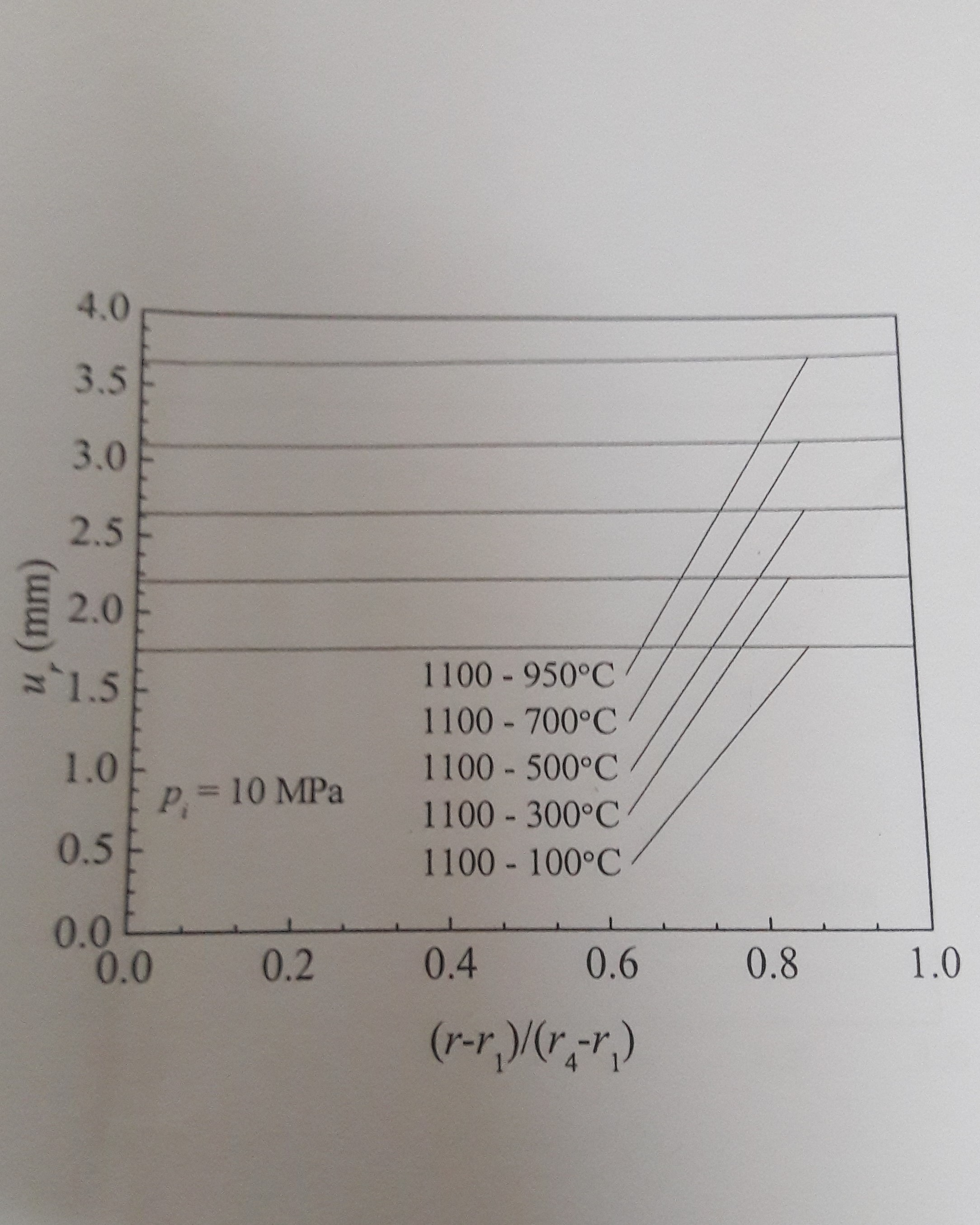This is the graph of deformation.So we can justify our assumptions by checking the graph of our simulated result with this graph too.The line 1100-100 means the inner line gets 1100*c temperature and the outer line of the cylinder gets 100*c temperature in all the graphs i have provided you so far.Will our model represent the similar graph of deformation sir? I have checked but i got a non linear curve line going upwards.

Sir you can take your time to help.I have bothered you much.Very Sorry for that.

Regards

• Dear peteroznewman,

Regards.

• If i provide the adhesive strength and,Hardness,Toughness and melting point then will it fulfill the demand for plastic properties?

The simplest way to create a material model that includes plasticity is to know the yield strength. With that you can add Bilinear Kinematic Hardening plasticity to a material that already has Isotropic Elasticity. Bilinear Kinematic Hardening requires one other value, the Tangent Modulus. This value describes the post-yield hardening behavior. If the Tangent Modulus is unknown, then a conservative approach is to set the Tangent Modulus to a value of zero. This creates what is known in textbooks as an Elastic Perfectly Plastic material.

Yield strength may be a function of temperature and you can input a table of temperature and yield strength. These might go up to the softening temperature, but at the melting temperature, the material is no longer a solid, but a liquid so there is no longer an elastic response to stress, there is a flow that is characterized by a material property called viscosity.

I can give the numerical solution of the graph (deformation vs distance) of this model.

When you say "this model", do you mean the model in the book that has the graph you are trying to match?  Yes, the tabular data of the output of the book model would be useful for adjusting parameters in an ANSYS model to try to match it.  However, what would be far more useful is the full description of the book model. A full description would be the geometry of all the layers, the material properties of all the layers, the temperature at which the layers are bonded together, the load history of the assembly and the conditions at the time the graph is plotted.

• I have attached the model i tried last time.

Put 10MPa pressure on the inner line of the cylinder and inner line temperature 1100 and outer line temperature 100.

And then teach me how can i get the residual stresses , deformation graph(like the above attached photo already).

• Here is a graph of radial displacement from your model in red averaging about 0.024 mm, while the value of the line for the 1000-100 condition that I read off the u plot from the book above is about 1.7 mm and I plotted that with a green line. The green line is a factor of about 70 times larger than the red line.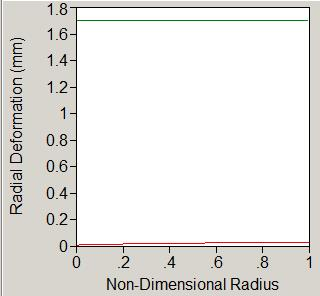The enormous difference between the value of the plot in the book and the value in your model is conclusive evidence that your model does not reproduce the physics that are in the book.

If you are interested in reproducing the results in the book with an ANSYS model, then you have to show for the numerical model in the book all of the following: the mathematical model, the assumptions made by the mathematical model, the inputs to the numerical model, the time-history of the materials in the numerical model.  Where does it say in the book that residual stresses are being plotted in the stress plot? I asked about the temperature during bonding of the layers. Where is that information?

• Thank you very much.I have understood you.

I will study what you have given me the doubts.Give some time to find out the answer of your demand.I will try to give the whole information what you asked me.

• Dear sir,

Can you please show me how to produce this graph from my model?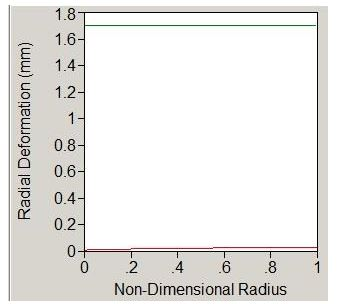And sir i will know you the other information as soon as possible.I am studying about your doubts.

• I used JMP to make the plot, but if you have Excel, you can make a similar plot.  Highlight the Tabular Data in the Deformation result for the Path, copy and paste into Excel.  Add a column to divide the path length by the maximum radius to create the Non-Dimensional Radius. Make a scatterplot of Radial Deformation vs Non-Dimensional Radius and add another Data Series in Excel that has the value 1.7 and you will have it.

• Sir,

" Add a column to divide the path length by the maximum radius to create the Non-Dimensional Radius. "

i haven't understood this line.

Another thing i tried to get the deformation to get a graph like you.But failed.I tried to get the  Radial Deformation vs Non-Dimensional Radius graph like this

..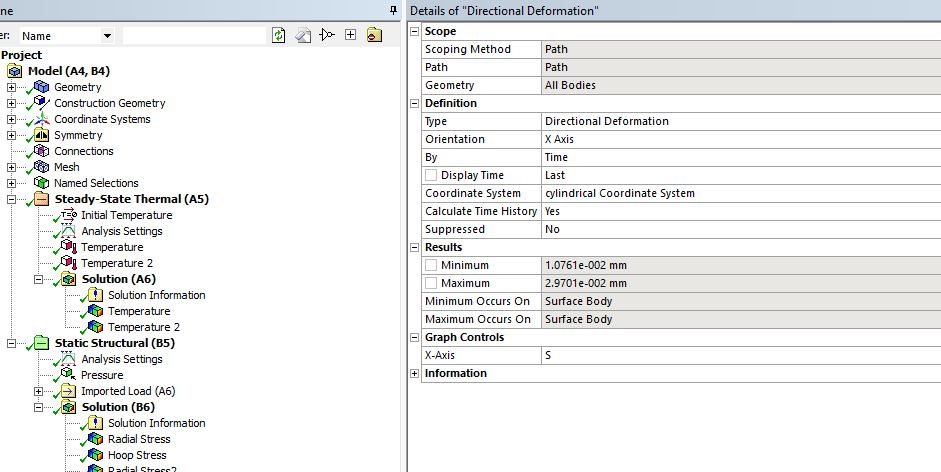But got this kind of graph which is like this curve going upwards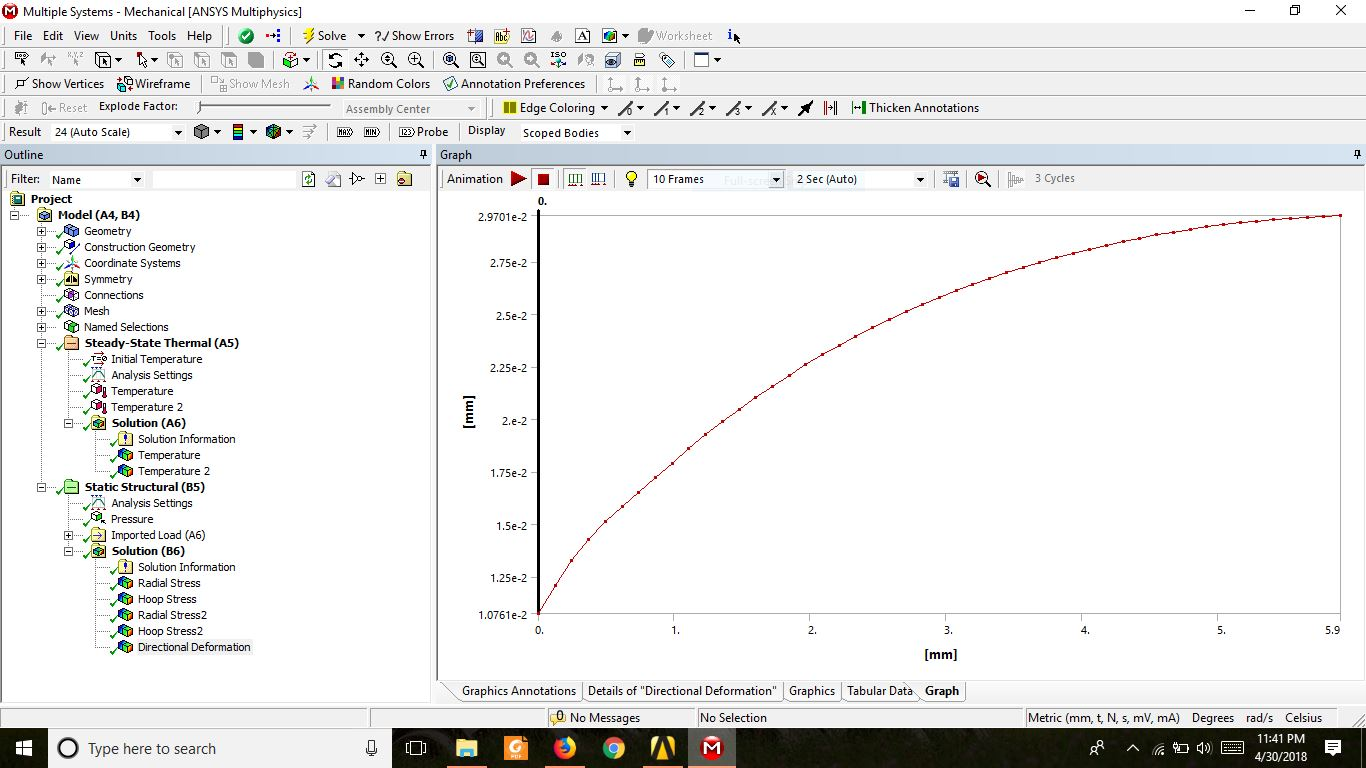• In Excel, insert column B and use a formula to divide column A by 5.9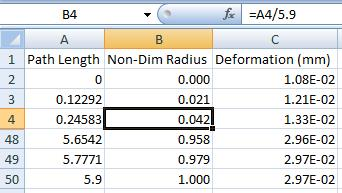Forget about the data curving up or down a little bit, the magnitude is wrong by a factor of 70.

• Thank you very much.

And i am studying what you told me to study.I will let you know my progress as soon as possible

Regards

• Dear sir,

Another issue has been risen out. If i want to make my solver understood that there will be no realtive displacement between each layer then how will i do it.

I meant i am getting the displacemnet graph as non linear.If i can determine that there is no relative displacement between each layer then the result should be a non linear graph.So how can i do it?

Regards

• There is no relative displacement between the layers because each layer shares the same node at the interface due to being in a single multibody part.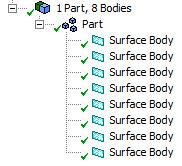• Dear peteroznewman,

I hope you are doing well.Hope you are enjoyoing your break.Sir do you have knowledge on mathematica? All the graph i have given to you solved by mathematica. So if you do have knowledge about that you can help me knowing the mathematical model, the assumptions made by the mathematical model, the inputs to the numerical model, the time-history of the materials in the numerical model.I will give you all the information to help me learning that sir.

Thanking you.

• Dear peteroznewman,

Sir i have changed the model to get a similar graph on mathematica. I have used mixture rule for the middle layer .Here you will get all the details about the model. I am attaching a pdf of the program. If you help me showing the video that how can i make this model on workbench(mechanical) .I will be grateful to you learning that.

Regards

• Sir

Have you understood the model sir?

Regards

• I had a first look at the code. Who wrote the code? There is often documentation that goes along with code. Is there any other supporting documentation for this code?

A conductivity material property, K2, is a function of radius:

K1 = 2.0; K3 = 11.7; (*watt/m^2c*)

K2[r_] := 1/(r2 - r3)*((K1 - K3)*r + (r2*K3 - r3*K1));

(*K1=2.0;*)

(*K2=K1;*)

(*K3=K1;*)

But commented out is a set of statements where K1, K2 and K3 all equal 2.0.

Is your goal to build an ANSYS model that reproduces the variable K2 as a function of radius?

Or is your goal to build an ANSYS model that reproduces the mathematica output when all values of K are equal to 2.0?

If you need conductivity as a function of radius, then you will have to implement Field Variables in ANSYS. Look at section 9.2 in the ANSYS Help.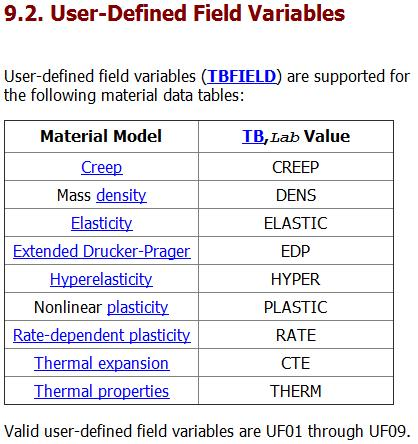• sir

The k2 of the model that means (k2)the middle layer of the model will be the variable of the radius.

Don't consider the commented portion sir. Sir can you help me showing the video of this model in ansys? As am i very new in ansys so i could have cleared my conceptions regarding this model sir.

Regards

• I have to learn how to create a material with a Field Variable, which will take some time.

In the mean time, you can see if you can get an ANSYS model to agree with the output of this program when all the material properties are a constant by commenting out the material variable properties for the second layer: K2, Alpha2, Nu2, and E2 and uncommenting the constant values for those properties.

What I have learned by reading the code is that it is using linear elastic material properties and there is no plasticity in the model.

I assume the radius values are in mm, and this code sets r1 = 226. The ANSYS models you provided had r1 = 4.1 mm. Make the mathematica code r1 = 4.1 to match this ANSYS model. Then the mathematica equations give us: r2 = 4.7, r3 = 5 and r4 = 6.6.  You provided a model called sss.wbpj that had three layers, but that model had r4 = 10.  Make mathematica Lstlayt = 5.0 to match this ANSYS geometry.

I know how to change 2D ANSYS models between Plane Stress and Plane Strain and the value of stress is different between those settings. You can look up the engineering equations that calculate stress for those two assumptions in a solid mechanics reference (book or Internet), and compare what you find with the Stress Calculation code in mathematica then show me in your reply which assumption has been programmed into mathematica: Plane Stress or Plane Strain.